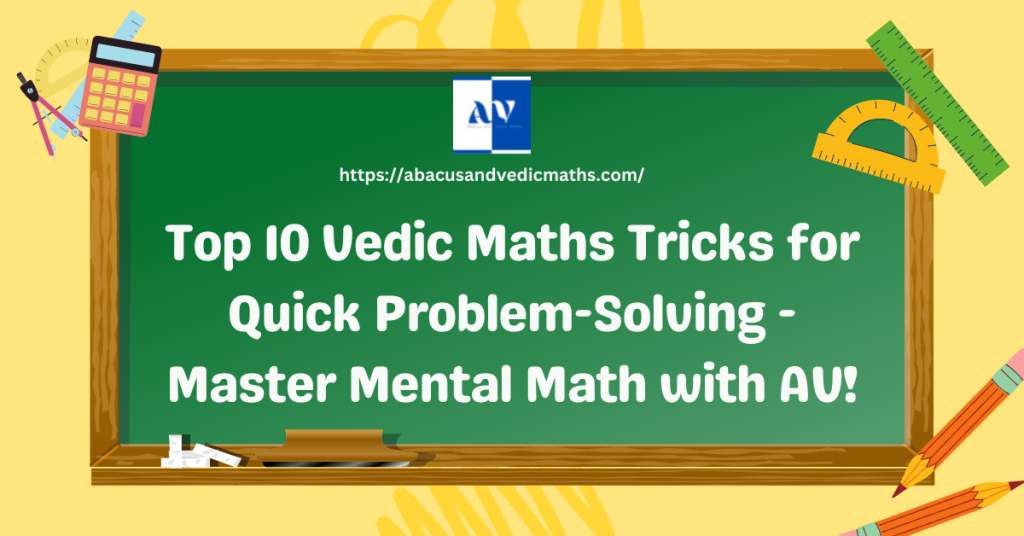Abacus and Vedic Maths# Top Ten Vedic Maths Tricks You Should Know for Fast Problem-Solving

Vedic Maths is well-known for its speedy and effective problem-solving techniques that can completely transform how you view mathematics. This blog presents the top ten Vedic Maths tricks to enhance mental math prowess and simplify complex calculations – from multiplication to division; these Vedic Maths tools will enable you to solve math issues swiftly and accurately.

Contents

## Multiplication by Vertically and Crosswise Multiplication

Explore the power of Vedic Maths’ vertical and crosswise multiplication technique that simplifies expanding two or more-digit numbers.## Squaring Numbers Ending in 5:

Use Vedic Maths’ trick of quickly squaring numbers ending in 5 to make mental calculations simpler and quicker. Multiplying Close to a Base: Simplify multiplication using Vedic Maths techniques that reduce complexity by multiplying numbers close to their bases.## Doubling and Halving Method:

Access this Vedic Maths technique designed to simplify multiplication and division.## Quick Division with Nikhilam Sutra:

Discover an alternative means for fast division of large numbers through Vedic Mathematics known as Nikhilam Sutra.## Finding Percentages with Ekadhikena Purvena:

Learn how the Ekadhikena Purvena method can quickly find percentages, saving time on calculations rapidly.## Checking Divisibility by Casting Out Nines:

Master this casting out nines technique to test divisibility quickly and detect errors in calculations rapidly.

## Discovering Fast Square Roots using Anurupyena Sutra:

Take advantage of Anurupyena Sutra to quickly locate square roots even for large numbers. Checking Fraction Equivalency using Antyayor Dasakepi: Discover this method to evaluate swiftly fraction equivalencies.

## Yavadunam Sutra to calculate cube roots

Use Yavadunam Sutra to calculate cube roots with conventional methods, saving time quickly.

## Conclusion:

With these top 10 Vedic Maths tricks, you can dramatically enhance your problem-solving skills and transform how you approach math. Leverage its speed and efficiency for fast mental calculations – enroll in our Vedic Maths program at AV to harness its incredible techniques!

At https://abacusandvedicmaths.com, learners interested in Vedic Maths programs such as those offered by Abacus & Vedicmaths (AV) can find more information and enroll.

Scroll to Top